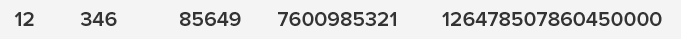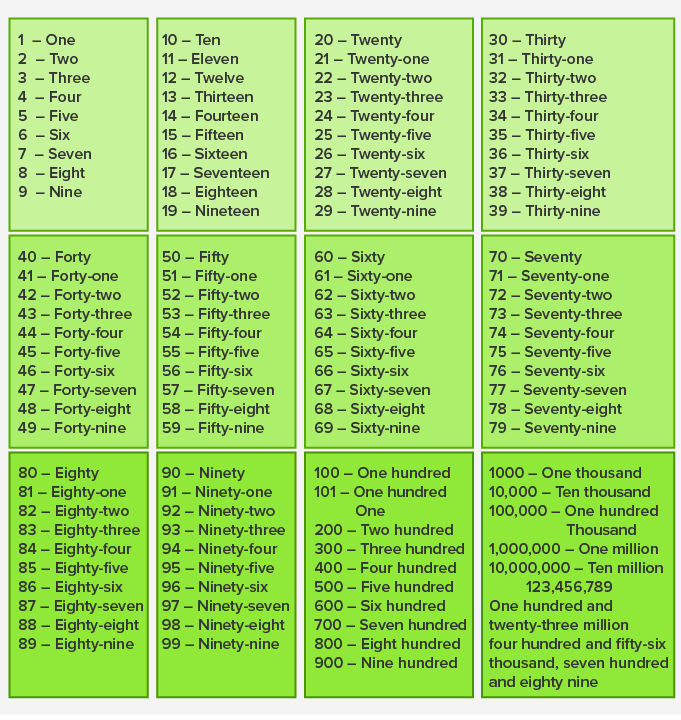# Cardinal Numbers - Definition with Examples

The Complete K-5 Math Learning Program Built for Your Child

• 40 Million Kids

Loved by kids and parent worldwide

• 50,000 Schools

Trusted by teachers across schools

• Comprehensive Curriculum

Aligned to Common Core

## Cardinal Numbers

Cardinal numbers are counting numbers. The numbers that we use for counting are called cardinal numbers.

Cardinal numbers tell us “How many?”

For example:

How many dogs are there in all?To know the total number of dogs, we need to count the dogs given in the image.10804Therefore, there are 8 dogs in all.

Example: How many kites are there in all?

Count the kites to know the total number of kites.On counting, we get:Thus, from the above examples, we see that to know “how many?” of something is there, we need to use cardinal numbers.

From where do cardinal numbers start?Cardinal numbers or counting numbers start from

How many cardinal numbers are there?

Cardinal numbers can go on and on and on. That means there are infinite counting numbers.

(Imagine, how many numbers you’ll need to count the number of stars in the sky or the number of sand grains in a desert?)

How are cardinal numbers formed?

The digits 1, 2, 3, 4, 5, 6, 7, 8, 9 and 0 are used to form several other cardinal numbers.

For example:Is zero (0) a cardinal number?

No, zero (0) is not a cardinal number. To know “how many” there should be something. Since 0 means nothing; it is not a cardinal number.

We can write cardinal numbers in numerals as 1, 2, 3, 4, and so on as well as in words like one, two, three, four, and so on.

The chart shows the cardinal numbers in figures as well in words.Cardinality

The cardinality of a group (set) tells how many objects or terms are there in that set or group.

Example: What is the cardinality of the flowers in the vase?

Here, there are 5 flowers in the vase. Therefore, the cardinality of flowers is 5.Cardinal numbers start from 1. Fractions and decimals represent a part (less than one) of a whole or a group. Therefore, fractions and decimals are not cardinal numbers.

 Fun Facts Cardinal numbers are also called natural numbers. Natural Numbers (Cardinal numbers) along with 0 form a set of whole numbers.
Won Numerous Awards & Honors